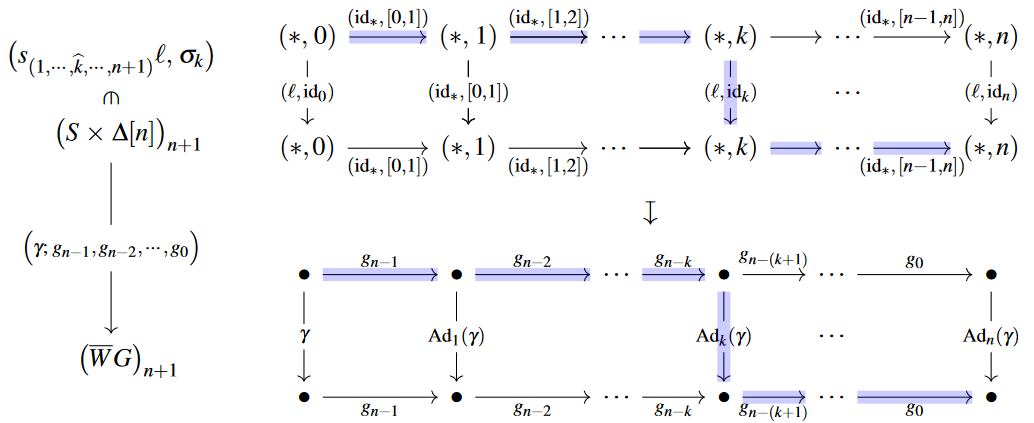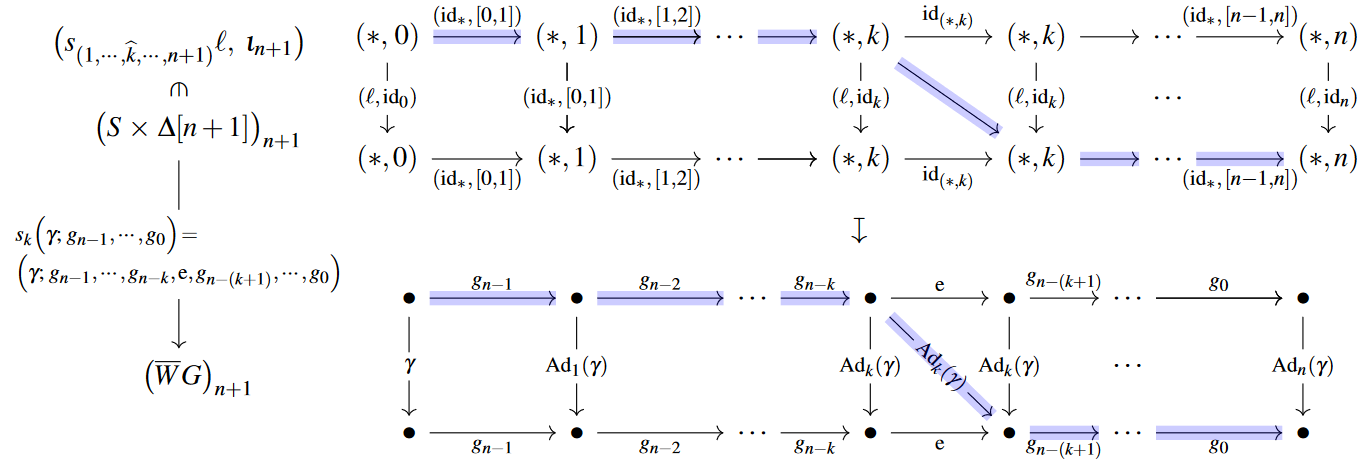# nLab function complex

Contents

### Context

#### Mapping spaces

internal hom/mapping space

# Contents

## Idea

In categories of simplicial objects, like SimplicialSets, the sSet-value hom-object between two objects is often called the function complex (“simplicial complex of functions from $X$ to $Y$).

The simplicial mapping complex or simplicial hom complex of simplicial function complex of two simplicial sets is the collection of maps, left homotopies and higher homotopies between them, all itself organized into a simplicial set.

More formally, SimplicialSets is a Cartesian monoidal category and the corresponding internal hom is what is traditionally known as the simplicial mapping complex.

## Definition

### General

Suppose $\mathcal{C}$ is a category that admits small coproducts.

Given simplicial objects $X,A\in \mathcal{C}^{\Delta^{op}}$, their function complex is the simplicial set

$Hom_{\Delta}(X,A) \;\; \in \;\; sSet$

whose set of $n$-simplices is the set of maps $\Delta^n\otimes A\to B$

$\big( Hom_{\Delta}(X,A) \big)_n \;\; = \;\; Hom_{\mathcal{C}} \big( \Delta^n \otimes X ,\, A \big) \,,$

where $\otimes$ denotes the copowering of simplicial objects over simplicial sets given by

$(S \otimes X)_n \,=\, \coprod_{i \in S_n} X_n \,.$

### In simplicial sets

Specializing the general definition above to $\mathcal{C} =$ sSet itself, the required tensoring is just the product of simplicial sets and so

###### Definition

The function complex between simplicial sets $X, A$ is the cartesian internal hom given by

$X, A \,\in\, sSet \;\;\;\; \vdash \;\;\;\; \left\{ \, \begin{array}{l} Maps(X,A) \;\; \in \;\; sSet \\ Maps(X,A)_\bullet \;\; = \;\; Hom_{sSet}\big( \Delta[\bullet] \times X ,\, A \big) \end{array} \right.$

(e.g. Goerss & Jardine (2009), I.5)

## Properties

### In simplicial sets

###### Proposition

(component formula for evaluation map on function complexes)
In SimplicialSets, the evaluation map of the function complex (from Def. ) — defined as the counit of the $\big(X \times (-)\big)\dashv Maps(X,-)$-adjunction — is given by:

$\begin{array}{cc} X \times Maps(X,A) &\xrightarrow{\;\; ev^X_A \;\;}& A \\ \phantom{A} \\ X_n \times \overset{ \mathclap{ Hom(X \times \Delta[n], A) } }{ \overbrace{ Maps(X,A)_n } } &\xrightarrow{\; \big(ev^X_A\big)_n \;}& A_n \\ \big( x ,\, f \big) &\mapsto& f(x, \iota_n) \,, \end{array}$

where

$\iota_n \;\coloneqq\; id_{\Delta[n]} \;\in\; Hom_{sSet}\big( \Delta[n],\, \Delta[n] \big) \;\simeq\; (\Delta[n])_n$

denotes the unique non-degenerate $n$-cell inside $\Delta[n]$.

(e.g. Goerss & Jardine (2009), p. 20)

###### Proposition

(simplicial nerve intertwines function complexes with functor categories)

Let $\mathcal{X}, \mathcal{A}$ be small strict categories and write $Maps(\mathcal{X}, \mathcal{A})$ for their functor category. Then under the simplicial nerve $N \;\colon\; Cat^{sm} \longrightarrow sSet$ this is isomorphic to the function complex (Def. ) between their separate simplicial nerves:

$N \big( Maps(\mathcal{X},\,\mathcal{A}) \big) \;\simeq\; Maps \big( N(\mathcal{X}) ,\, N(\mathcal{A}) \big) \,.$

(For the proof see here at nerve.)

## Examples

### General

###### Example

(simplicial mapping complex between nerves of groupoids) For $N(\mathcal{G}_i)$ the nerves of groupoids, their simplicial mapping complex is isomorphic to the nerve of the functor groupoid from $\mathcal{G}_1$ to $\mathcal{G}_2$:

$\big[ N(\mathcal{G}_1), \, N(\mathcal{G}_2) \big] \;\; \simeq \;\; N \big( Func(\mathcal{G}_1, \, \mathcal{G}_2) \big) \;\;\; \in \; SimplicialSets$

### Nerves of inertia groupoids

###### Example

(evaluation map on function complexes which are nerves of inertia groupoids)

Consider a discrete group $G$. We write $\mathbf{B}G$ for its delooping groupoid, here specifically for its strict version $\mathbf{B}G \,\coloneqq\,\big(G \rightrightarrows \ast \big)$. The nerve of this groupoid is the canonical model for the simplicial classifying space: $N \mathbf{B}G \,=\, \overline{W}G \,.$ The functor groupoid

$\Lambda \mathbf{B}G \;\coloneqq\; Maps\big( \mathbf{B}\mathbb{Z} ,\, \mathbf{B}G \big)$

is also known as the inertia groupoid of $\mathbf{B}G$.

The simplicial nerve of this functor groupoid is the following function complex of simplicial sets (using Prop. ):

$N \Lambda \mathbf{B}G \;\coloneqq\; Maps\big( N \mathbf{B}\mathbb{Z} ,\, N \mathbf{B}G \big) \;\simeq\; Maps\big( S ,\, N \mathbf{B}G \big) \,.$

Here in the second step we introduced the simplicial set

(1)$S \;\coloneqq\; \Delta/\partial \Delta \;\;\; \in \; sSet \,,$

which is the minimal model for $\mathbf{B}\mathbb{Z}$, whose unique non-degenerate 1-cell we will denote by

$\ell \;\coloneqq\; [0,1] \in \Delta_1 \twoheadrightarrow S_1$

We now describe in detail the evaluation map on the simplicial function complex $Maps\big( S ,\, N \mathbf{B}G \big) \,.$

To that end, we denote by

(2)$\big( \gamma ;\, g_{n-1} ,\, g_{n-2} ,\, \cdots ,\, g_{0} \big) \;\; \in \;\; \mathrm{Hom} \big( S \times \Delta[n] ,\, \overline{W}G \big) \;\simeq\; \Big( N \mathrm{Map} \big( { \mathbf{B}\mathbb{Z} } ,\, { \mathbf{B}G } \big) \Big)_{n}$

the $n$-cell in the nerve of the inertia groupoid that corresponds to the sequence of natural transformation which start at the functor

$\gamma \,\in\, G \,\simeq\, \mathrm{Hom}_{\mathrm{Grp}}(\mathbb{Z}, G) \,\simeq\, \mathrm{Hom}\big( \mathbf{B}\mathbb{Z} ,\, \mathbf{B}G \big)$

and successively have components $g_{n-\bullet} \in G$.

Throughout we are writing “$Hom$” for hom-sets and “$Map$” for hom-objects, i.e. for internal homs and we keep tacitly going back and forth through the bijections in (2).

If we abbreviate (this follows conventions familiar in discussion of transgression in group cohomology)

\begin{aligned} Ad_{k}(\gamma) & \;\coloneqq\; Ad_{(g_{n-1}\cdots g_{n-k})}(\gamma) \\ & \;\coloneqq\; (g_{n-1} \cdots g_{n-k})^{-1} \cdot \gamma \cdot (g_{n-1} \cdots g_{n-k}) \,, \end{aligned}

then this corresponds to a sequence of composable morphisms in the inertia groupoid of this form:

$\gamma \xrightarrow{ g_{n-1} } Ad_{1}(\gamma) \xrightarrow{ g_{n-2} } \cdots \xrightarrow{\; g_0 \;} Ad_{n}(\gamma) \;\;\;\;\; \in \;\; \Lambda B G$

The simplicial map (2) maps the non-degenerate $(n+1)$-cells in the product of simplicial sets (see the discussion there) of $S$ (1) with the simplicial $n$-simplex as follows:This is not exactly what we need unwinding the evaluation map, but it is close: the image of (2) under the $k$th degeneracy map evidently gives the following mapping:This is the type of mapping that appears in the component formula for the evaluation map on function complexes (from Prop. ). Hence we find that this evaluation map is given on non-degenerate $(n+1)$-simplices in the product (see again there) as follows:

The expressions on the right happen to be those that appear in the formula for transgression in group cohomology, see there.

The original definition of a function complex in the generality stated above:

• Daniel M. Kan, On c.s.s. categories, Boletín de la Sociedad Matemática Mexicana 2 (1957), 82–94. PDF.

In function complxes in the generality of simplicial presheaves

Textbook account in simplicial homotopy theory:

Introduction with an eye towards quasicategories: